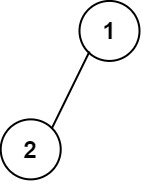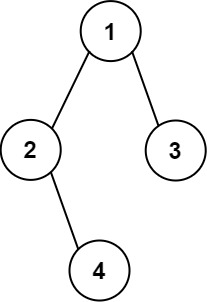# GeetCode Hub

Given the `root` of a binary tree, construct a 0-indexed `m x n` string matrix `res` that represents a formatted layout of the tree. The formatted layout matrix should be constructed using the following rules:

• The height of the tree is `height` and the number of rows `m` should be equal to `height + 1`.
• The number of columns `n` should be equal to `2height+1 - 1`.
• Place the root node in the middle of the top row (more formally, at location `res[(n-1)/2]`).
• For each node that has been placed in the matrix at position `res[r][c]`, place its left child at `res[r+1][c-2height-r-1]` and its right child at `res[r+1][c+2height-r-1]`.
• Continue this process until all the nodes in the tree have been placed.
• Any empty cells should contain the empty string `""`.

Return the constructed matrix `res`.

Example 1:```Input: root = [1,2]
Output:
[["","1",""],
["2","",""]]
```

Example 2:```Input: root = [1,2,3,null,4]
Output:
[["","","","1","","",""],
["","2","","","","3",""],
["","","4","","","",""]]
```

Constraints:

• The number of nodes in the tree is in the range `[1, 210]`.
• `-99 <= Node.val <= 99`
• The depth of the tree will be in the range `[1, 10]`.

/** * Definition for a binary tree node. * public class TreeNode { * int val; * TreeNode left; * TreeNode right; * TreeNode() {} * TreeNode(int val) { this.val = val; } * TreeNode(int val, TreeNode left, TreeNode right) { * this.val = val; * this.left = left; * this.right = right; * } * } */ class Solution { public List<List<String>> printTree(TreeNode root) { } }